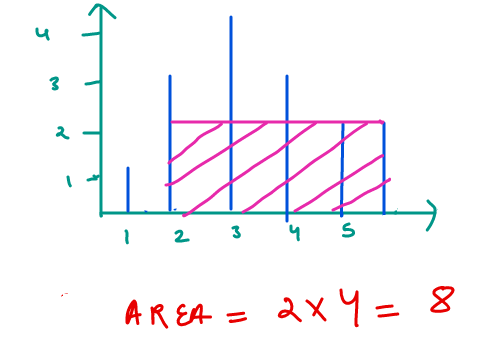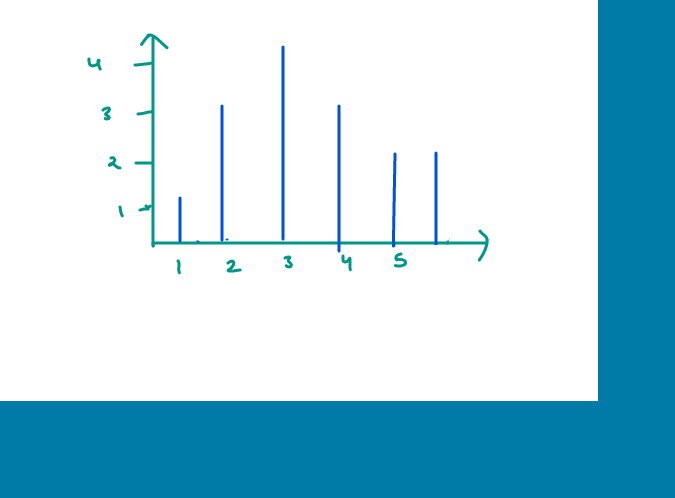# Container with most water

Given n positive integers ( ` a`0`, a`1`, a`2`, ... a`n-1) , a straight vertical line is drawn from coordinates `(i, a`i`)` till ` (i, 0) `.
Find two lines, which together with x-axis forms a container, such that the container contains the most water.
The program should return an integer which corresponds to the maximum area of water that can be contained.

#### Example Test Cases

##### Sample Test Case 1

Input Array: ` 1 3 4 3 2 2 `
Expected Output: ` 8 `
Explanation: Maximum water will be trapped b/w index 1 and indice 5. Take a look at the image below.#### Solution

Since the area occupied b/w any two lines `a`i and `a`j is `(j - i)*min(a`i`, a`j`)`.
We will try to maximise both the ` (j - i) ` and ` min(a`i`, a`j`)`
The maximum value of ` j - i ` is ` n - 1 ` when ` i = 0 ` and ` j = n - 1`and for that combination possible area value will be ` (n-1)*min(a`0`, a`n-1`)`.

Now, if we want to find a better solution than that, we will have to reduce the width (which may or may not lead to increase in the height). Since, we want to maximize the height, we will remove the line with lower height. So if `a`0` < a`n-1, we will reduce the width by 1 from left, otherwise we will reduce the width from right. We will keep following this procedure until we can’t reduce width fuurther. The maximum of all these values will be our answer. See the below images for the visualization of the above procedure:#### Implementation

```#include <iostream>
#include <vector>
using namespace std;

int maxArea(vector<int>& height) {
int n = height.size();
int current = -1;
int left = 0, right = n-1;
while (left < right) {
int possibleSum = min(height[left], height[right])*(right - left);
current = max(current, possibleSum);
if (height[left] < height[right]) {
left++;
}
else {
right--;
}
}
return current;
}

int main() {
vector<int> height = {1, 3, 4, 3, 2, 2};
cout << "Max area = " << maxArea(height) << "\n";
return 0;
}```
Scroll to Top
[gravityforms id="5" description="false" titla="false" ajax="true"]